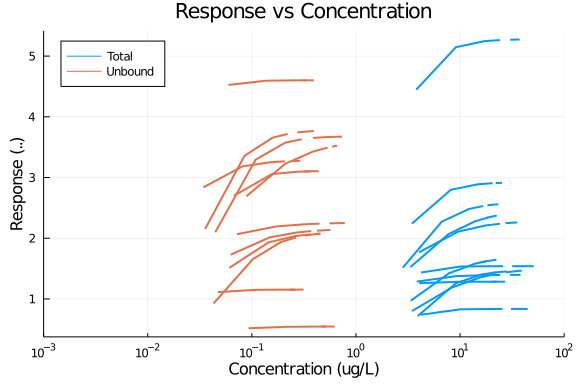# Exercise 50 - Analysis of multiple subjects concentration and response-time profiles

### Background

* Structural model - Two compartment Model
* Route of administration - IV Infusion
* Dosage Regimen - 566 μg
* Number of Subjects - 12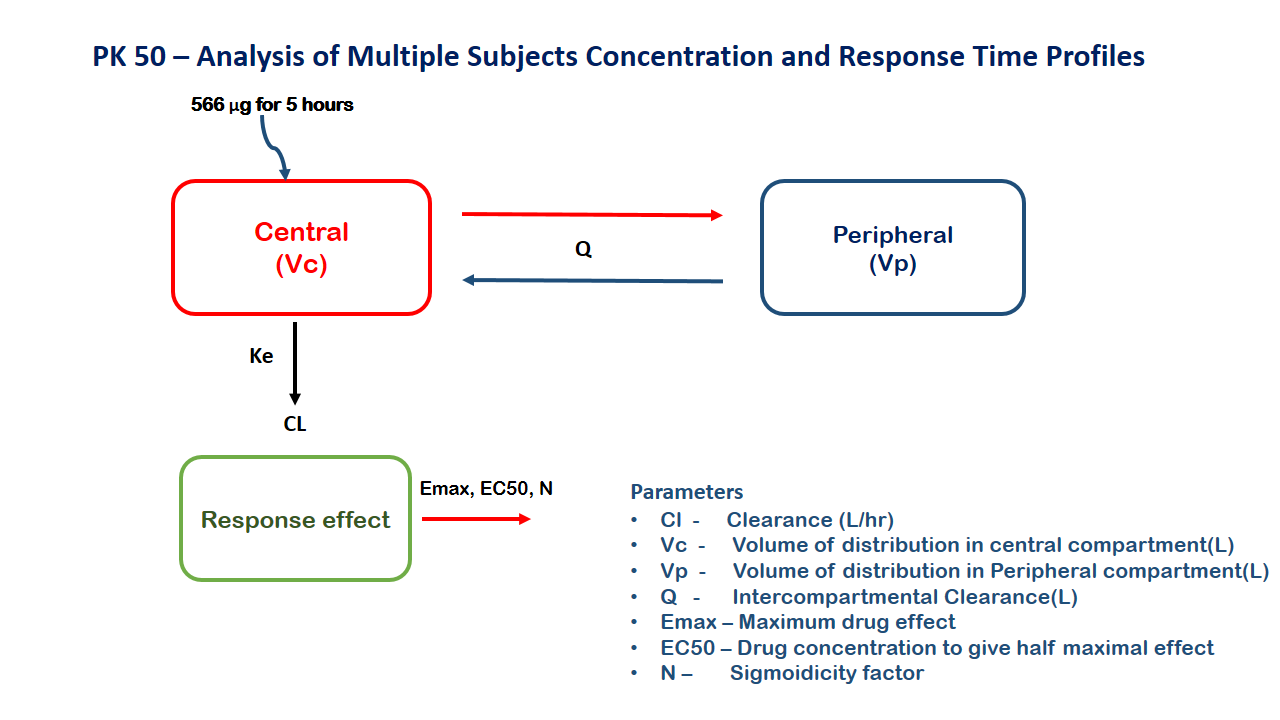### Learning Outcome

To analyze and interpret exposure and effect with plasma protein binding as a co-covariate of pk parameters and exposure

### Objectives

To build a sequential PKPD model for a drug considering fraction unbound as a covariate

### Libraries

call the "necessary" libraries to get started.

using Pumas
using Plots
using CSV
using StatsPlots
using Random


### Model

To build a sequential two compartment PKPD model for a drug after infusion over 5 hours

pk_50           = @model begin
@param begin
tvcl        ∈ RealDomain(lower=0)
tvcld       ∈ RealDomain(lower=0)
tvvc        ∈ RealDomain(lower=0)
tvvt        ∈ RealDomain(lower=0)
tvec50      ∈ RealDomain(lower=0)
tvemax      ∈ RealDomain(lower=0)
tvsigma     ∈ RealDomain(lower=0)
Ω           ∈ PDiagDomain(7)
σ_prop      ∈ RealDomain(lower=0)
σ_add       ∈ RealDomain(lower=0)
end

@random begin
η           ~ MvNormal(Ω)
end

@covariates fu

@pre begin
Cl          = tvcl * (1/fu) * exp(η)
Cld         = tvcld * (1/fu) * exp(η)
Vc          = tvvc * (1/fu) * exp(η)
Vt          = tvvt * (1/fu) * exp(η)
EC50        = tvec50 * (fu) * exp(η)
Emax        = tvemax * exp(η)
sigma       = tvsigma * exp(η)
end

@dynamics begin
Central'    = -(Cl/Vc)*Central - (Cld/Vc)*Central + (Cld/Vt)*Peripheral
Peripheral' = (Cld/Vc)*Central - (Cld/Vt)*Peripheral
end

@derived begin
cp          = @. Central/Vc
dv_cp       ~ @. Normal(cp, sqrt(cp^2*σ_prop))
ef          = @. Emax*(cp^sigma)/(EC50^sigma+cp^sigma)
dv_ef       ~ @. Normal(ef, σ_add)
end
end

PumasModel
Parameters: tvcl, tvcld, tvvc, tvvt, tvec50, tvemax, tvsigma, Ω, σ_prop,
σ_add
Random effects: η
Covariates: fu
Dynamical variables: Central, Peripheral
Derived: cp, dv_cp, ef, dv_ef
Observed: cp, dv_cp, ef, dv_ef


### Parameters

Parameters provided for simulation are as below. tv represents the typical value for parameters.

• Cl - Clearance (L/hr)

• Vc - Volume of distribution in central compartment (L)

• Vp - Volume of distribution in Peripheral compartment (L)

• Q - Intercompartmental Clearance (L/hr)

• EC50 - Concentration which produces 50% effect (μg/L)

• Emax - Maximum Effect

• sigma - Sigmoidicity factor

param = (tvcl    = 11.4,
tvcld   = 4.35,
tvvc    = 19.9,
tvvt    = 30.9,
tvec50  = 1.8,
tvemax  = 2.1,
tvsigma = 2.1,
Ω       = Diagonal([0.0784,0.1521,0.0841,0.1225,0.16,0.36,0.09]),
σ_prop  = 0.00,
σ_add   = 0.00)

(tvcl = 11.4, tvcld = 4.35, tvvc = 19.9, tvvt = 30.9, tvec50 = 1.8, tvemax
= 2.1, tvsigma = 2.1, Ω = [0.0784 0.0 … 0.0 0.0; 0.0 0.1521 … 0.0 0.0; … ;
0.0 0.0 … 0.36 0.0; 0.0 0.0 … 0.0 0.09], σ_prop = 0.0, σ_add = 0.0)


### Dosage Regimen

A group of 12 subjects are administered a dose of 566 μg infused over 5 hours

## Total Plasma Concentration
ev1       = DosageRegimen(566, cmt=1, time=0, duration=5)
sub_total = map(i -> Subject(id=i, events=ev1, covariates=(fu=1,group="Total")), 1:12)

## Unbound Plasma Concentration
fu1 = Normal(0.016,0.0049)
Random.seed!(1234)
fu  = rand(fu1, 12)

df_unbound    = map(((i,fui),) -> DataFrame(id=i, amt=566, time=0, cmt=1, evid=1, rate=113.2, dv_cp=missing, dv_ef=missing, fu=fui, group="Unbound"), zip(1:12, fu))
df1_unbound   = vcat(DataFrame.(df_unbound)...)
pop12_unbound = read_pumas(df1_unbound, observations=[:dv_cp, :dv_ef], covariates=[:fu, :group])

pop24_sub = [sub_total;pop12_unbound]

Population
Subjects: 24
Covariates: fu, group


### Simulation

Simulate the data and create a dataframe with specific data points.

Random.seed!(123)
sim_pop24_sub = simobs(pk_50, pop24_sub, param, obstimes=[0,0.25,0.5,0.75,1,2,3,4,4.999,5.03,5.08,5.17,5.25,5.5,5.75,6,6.5,7,8,9,10,12,24])
df1           = DataFrame(sim_pop24_sub)


### Plot

Plot a graph of Concentrations vs Time

@df df1 plot(:time, :cp, yaxis=:log, group=:group,
title="Concentration vs Time", xlabel="Time (hrs)", ylabel="Concentration (ug/L)",
xticks=[0,5,10,15,20,25], xlims=(0,25), linewidth=2,
yticks=[0.001,0.01,0.1,1,10,100], ylims=(0.001,100))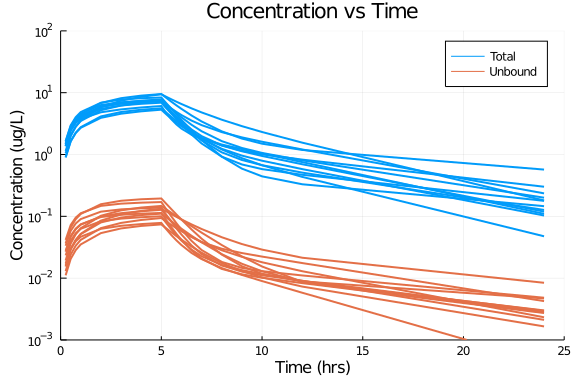Plot a graph of Response vs Concentrations

@df df1 plot(:cp, :ef, xaxis=:log, group=:group, linewidth=2,
title="Response vs Concentration", xlabel="Concentration (ug/L)", ylabel="Response (..)",
xticks=[0.001,0.01,0.1,1,10,100], xlims=(0.001,100))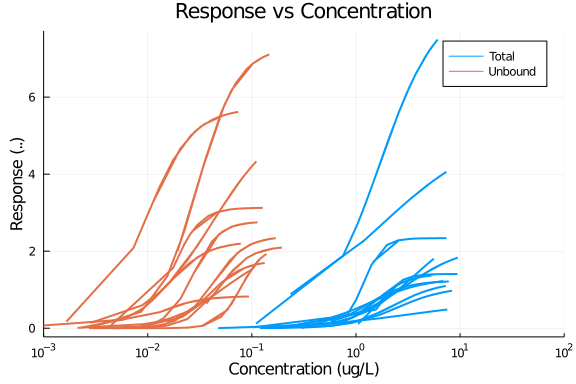Question - 1 and 2

1. What infusion rate do you aim at in the present patient population during the first hour to reach a plasma concentration > 10 μg/L and < 50 μg/L.

Ans: We have targeted a plasma concentration of 30 μg/L and thus the dose required to achieve those concentration is 784 μg given as an IV-infusion over 1 hour followed by a 7843 μg given as an IV-infusion over 23 hours

1. What Infusion rate is needed to remain at the staedy state plasma concentration between 1 and 24 hours?

Ans: An infusion rate of 341 μg/hr is given to achieve the steady-state plasma concentration of 30 μg/L

##Dosage Regimen - Total Plasma Concentration
ev12  = DosageRegimen([784,7843], cmt=1, time=[0,1], rate=[784,341])
pop12 = Population(map(i -> Subject(id=i, events=ev12, covariates=(fu=1,group="Total")), 1:12))

##Simulation
Random.seed!(123)
sim12 = simobs(pk_50, pop12, param, obstimes=[0,0.25,0.5,0.75,1,2,3,4,4.999,5.03,5.08,5.17,5.25,5.5,5.75,6,6.5,7,8,9,10,12,24])
df12  = DataFrame(sim12)
dropmissing!(df12, :cp)

@df df12 plot(:time, :cp, group=:id,
title="Concentration vs Time", xlabel="Time (hrs)", ylabel="Concentration (ug/L)",
xticks=[0,5,10,15,20,25], xlims=(0,25), linewidth=2, label=false)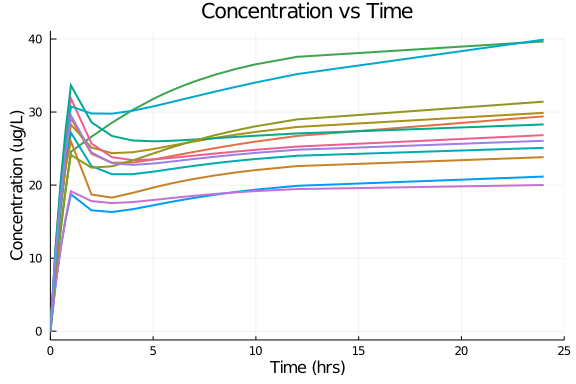@df df12 plot(:time, :cp, yaxis=:log, group=:id,
title="Concentration vs Time", xlabel="Time (hrs)", ylabel="Concentration (ug/L)",
xticks=[0,5,10,15,20,25], xlims=(0,25), linewidth=2, label=false,
yticks=[0.001,0.01,0.1,1,10,100], ylims=(0.001,100))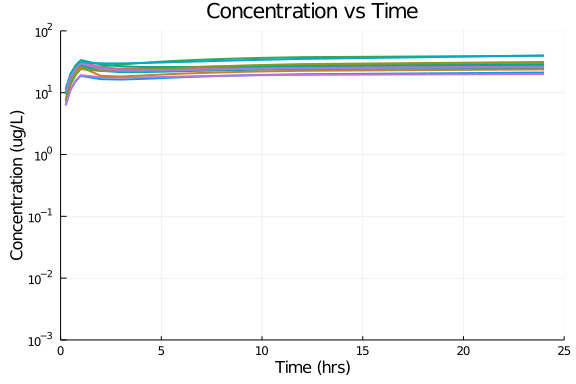Question - 3

1. What unbound plasma concentration are reached (given the range) with the infusion rates calculated for the 1+23 hrs regimen? How does the variability seen in the predcited exposure at 1 and 24 hours compare between total and unbound concentration?

## Dosage Regimen - Unbound Plasma Concentration
df_3  = map(((i,fui),) -> DataFrame(id=i, amt=[784,7800], time=[0,1], cmt=[1,1], evid=[1,1], rate=[784,339], dv_cp=missing, dv_ef=missing, fu=fui, group="Unbound"), zip(1:12, fu))
df1_3 = vcat(DataFrame.(df_3)...)
pop_3_unbound = read_pumas(df1_3, observations=[:dv_cp, :dv_ef], covariates=[:fu, :group])

pop_3   = [pop12;pop_3_unbound]

## Simulation
Random.seed!(12345)
sim3 = simobs(pk_50, pop_3, param, obstimes=[0.1,0.25,0.5,0.75,1.0,2,3,4,4.999,5.03,5.08,5.17,5.25,5.5,5.75,6,6.5,7,8,9,10,12,24])
df_sim3 = DataFrame(sim3)

@df df_sim3 plot(:time, :cp, yaxis=:log, group=:group,
title="Concentration vs Time", xlabel="Time (hrs)", ylabel="Concentration (ug/L)",
xticks=[0,5,10,15,20,25], xlims=(0,25), linewidth=2, label=false,
yticks=[0.001,0.01,0.1,1,10,100], ylims=(0.001,100))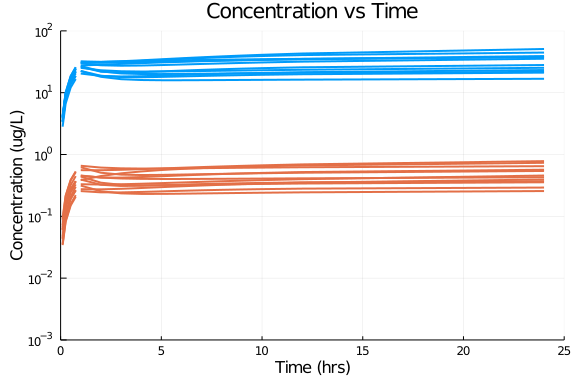Question - 4

1. What exposure is needed in the new 1 + 23 hours infusion study to establish a response greater than one(1) Response unit?

Ans: The new 1 + 23 hr infusion chosen to achieve a steady-state Concentration of 30 μg/L will help to achieve repsonse greater than 1 unit.

@df df_sim3 plot(:cp, :ef, group=:group, xaxis=:log, legend=:topleft, linewidth=2,
title="Response vs Concentration", xlabel="Concentration (ug/L)", ylabel="Response (..)",
xticks=[0.001,0.01,0.1,1,10,100], xlims=(0.001,100))